# 输电线路鸟巢识别中的无人机优化巡检研究Research on Optimizing UAV Inspection for Transmission Line Bird-Nest Detection

DOI: 10.12677/AIRR.2020.92013, PDF, HTML, XML, 下载: 94  浏览: 738  科研立项经费支持

Abstract: Bird damage is one of the critical factors which threaten the stability of China’s electric transmission lines. Analyzing the increasing frequency of transmission malfunction owing to birds in recent years, this article is propounding an optimizing principle for UAV (unmanned aerial vehicles) inspection in bird nest detection. For identifying towers, Hough arithmetic is adopted to extract features from UAV images. In these tower-identified areas, through extracting color and texture features, bird nest is recognizable. Moreover, in allusion to inspection omission, SolidWorks contributes to constructing three-dimensional simulation models of umbrella-type high-tension towers and bird nests. Therefore, a type of UAV shooting rule is refined to detect nests, which is capable of effectively lowering towers’ disruption to test bird nests, and consequently, boosting detection sensitivity.

1. 引言

2. 鸟巢识别策略Figure 1. Flow chart of bird nest identification strategy

2.1. Hough算法识别塔杆区域

Hough算法是一种经典的直线检测方法，该算法的核心思想是构造一种从图像空间到参数空间的映射关系。其映射关系公式如下：

$\left(x,y\right)\to \rho =x\mathrm{cos}\theta +y\mathrm{sin}\theta \text{ }\text{ }\text{ }\left(0\le \theta <2\pi \right)$ (1)

Hough算法的实现步骤：

1) 参数空间离散化，对每个参数空间的单元赋予一个初始值为“0”的累加器；

2) 若某条正弦曲线恰好经过参数空间单元，则该单元累加器的值就加1；

3) 遍历直角坐标系中的所有点后，检验参数空间中每个累加器的值。

1) 若第i分块内坐标数量 ${P}_{i}$ 均大于等于6，则该分块是塔杆区域；

2) 若第i分块内坐标数量 ${P}_{i}$ 均小于6，则该分块是非塔杆区域。

3) 对所有分块进行分析，如果非塔杆区域数目大于总的分块数目的70%，则该巡检图像不符合检测条件；

4) 若巡检图像符合准则3)，则合并塔杆区域，并确定塔杆区域的位置。

2.2. 基于颜色与纹理特征的鸟巢检测

2.2.1. 颜色特征检测

20世纪末，Androutsos   等人通过实验对HSV颜色空间进行了大致划分，亮度大于75%并且饱和度大于20%为彩色区域，亮度小于25%为黑色区域，亮度大于75%且饱和度小于20%为白色区域，其他为彩色区域。文献  根据人的视觉分辨能力，把色调H空间分为8份，饱和度S分为4份，亮度V空间分为3份，故HSV颜色空间可以划分为：

$H:\left\{\begin{array}{l}0\text{ }\text{ }\text{ }\text{ }\text{ }\text{ }\text{ }\text{ }\text{ }\text{ }H\in \left[224,20\right]\\ 1\text{ }\text{ }\text{ }\text{ }\text{ }\text{ }\text{ }\text{ }\text{ }\text{ }\text{ }H\in \left[20,28\right]\\ 2\text{ }\text{ }\text{ }\text{ }\text{ }\text{ }\text{ }\text{ }\text{ }\text{ }H\in \left[29,53\right]\\ 3\text{ }\text{ }\text{ }\text{ }\text{ }\text{ }\text{ }\text{ }\text{ }\text{ }H\in \left[54,110\right]\\ 4\text{ }\text{ }\text{ }\text{ }\text{ }\text{ }\text{ }\text{ }\text{ }\text{ }H\in \left[111,135\right]\\ 5\text{ }\text{ }\text{ }\text{ }\text{ }\text{ }\text{ }\text{ }\text{ }\text{ }H\in \left[136,192\right]\\ 6\text{ }\text{ }\text{ }\text{ }\text{ }\text{ }\text{ }\text{ }\text{ }\text{ }H\in \left[193,210\right]\\ 7\text{ }\text{ }\text{ }\text{ }\text{ }\text{ }\text{ }\text{ }\text{ }\text{ }H\in \left[211,223\right]\end{array}\text{ }\text{ }\text{ }\text{ }S:\left\{\begin{array}{l}0\text{ }\text{ }\text{ }\text{ }\text{ }\text{ }\text{ }\text{ }\text{ }\text{ }S\in \left[0.00,0.15\right]\\ 1\text{ }\text{ }\text{ }\text{ }\text{ }\text{ }\text{ }\text{ }\text{ }\text{ }\text{ }S\in \left[0.16,0.35\right]\\ 2\text{ }\text{ }\text{ }\text{ }\text{ }\text{ }\text{ }\text{ }\text{ }S\in \left[0.36,0.70\right]\\ 3\text{ }\text{ }\text{ }\text{ }\text{ }\text{ }\text{ }\text{ }\text{ }\text{ }S\in \left[0.71,1.00\right]\end{array}\text{ }\text{ }\text{ }\text{ }V:\left\{\begin{array}{l}0\text{ }\text{ }\text{ }\text{ }\text{ }\text{ }\text{ }\text{ }\text{ }\text{ }V\in \left[0,0.2\right]\\ 1\text{ }\text{ }\text{ }\text{ }\text{ }\text{ }\text{ }\text{ }\text{ }\text{ }\text{ }V\in \left[0.2,0.7\right]\\ 2\text{ }\text{ }\text{ }\text{ }\text{ }\text{ }\text{ }\text{ }\text{ }V\in \left[0.71,1\right]\end{array}$ (2)

2.2.2. 纹理特征检测

${T}_{e}={\left[{Q}_{1},{Q}_{2},{Q}_{3},{Q}_{4}\right]}^{\text{T}}$ (3)

${T}_{e\text{_Standard}}={\left[{Q}_{S1},{Q}_{S2},{Q}_{S3},{Q}_{S4}\right]}^{\text{T}}$ (4)

${T}_{e\text{_Price}}=W×{T}_{e}$ (5)

${T}_{e\text{_Sta_Price}}=W×{T}_{e\text{_Standard}}$ (6)

${d}_{if}=|\frac{{T}_{e\text{_Price}}-{T}_{e\text{_Sta_Price}}}{{T}_{e\text{_Price}}+{T}_{e\text{_Sta_Price}}}|$ (7)

a. 标准纹理向量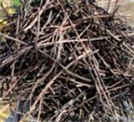(a)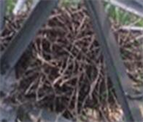(b)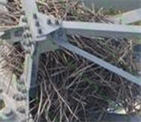(c)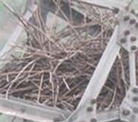(d)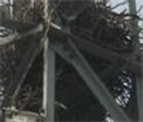(e)

Figure 2. Standard nest image group

${T}_{e\text{_Standard}}={\left[0.0539,3.6528,1.3893,0.1527\right]}^{\text{T}}$

b. 权重向量

${w}_{j}=\frac{1/{Q}_{j}}{\underset{i=1}{\overset{4}{\sum }}1/{Q}_{i}},\text{ }\text{ }j=1,2,3,4$ (8)

$W=\left[0.5326,0.0384,00361,0.3829\right]$.

${T}_{e\text{_Sta_Price}}=W×{T}_{e\text{_standard}}=0.2791$.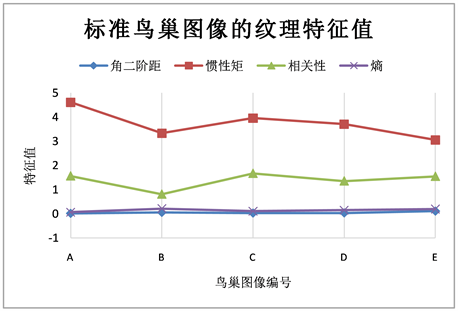Figure 3. Texture feature value of standard bird’s nest image

3. 拍摄角度的确定及平行准则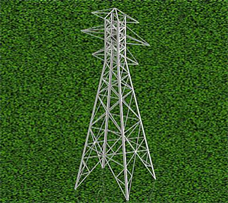(a)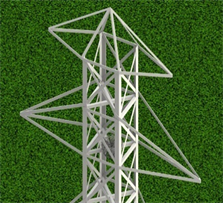(b)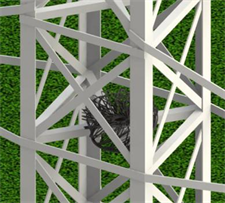(c)

Figure 4. Simulation diagram

$\Phi =\frac{k}{{d}_{if}}$ (9)

4. 实验验证与结果分析

4.1. 鸟巢识别率Table 1. Test results of transmission line tower bird’s nest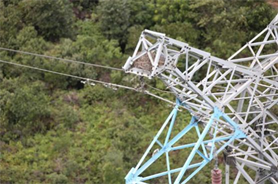Figure 5. Schematic diagram of test results

4.2. 无人机拍摄角度的确定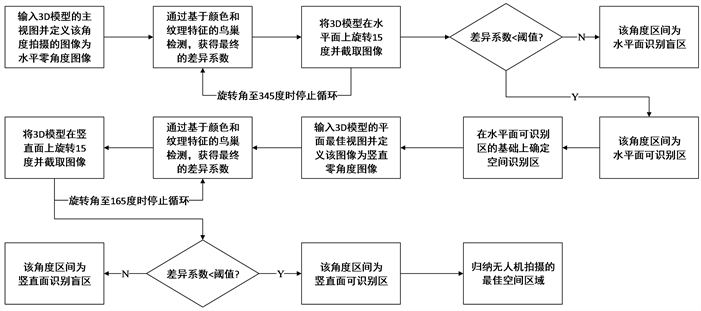Figure 6. Flow chart of determining shooting angle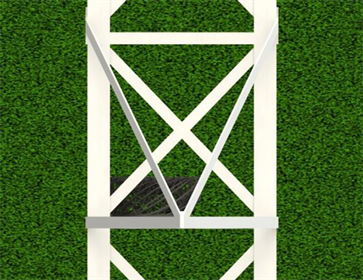Figure 7. Horizontal zero angle diagram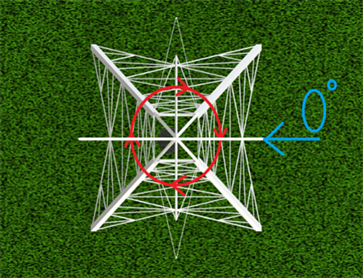Figure 8. Model rotation diagramTable 2. Statistical table of horizontal shooting angle and final difference coefficient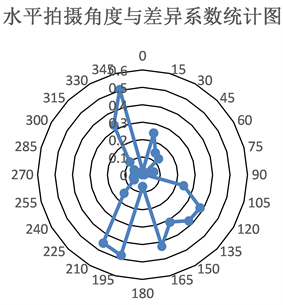Figure 9. Statistical chart of shooting angle and difference coefficient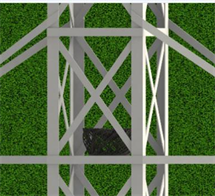(a)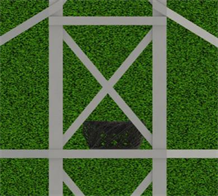(b)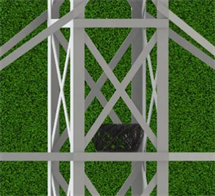(c)

Figure 10. Schematic diagram of bird’s nest horizontal shooting angle

a. 竖直面拍摄角度的确定Table 3. Statistical table of vertical shooting angle and final difference coefficient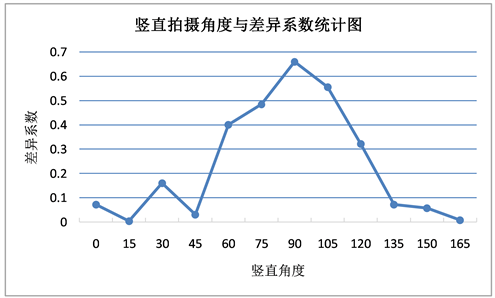Figure 11. Statistical chart of vertical shooting angle and difference coefficient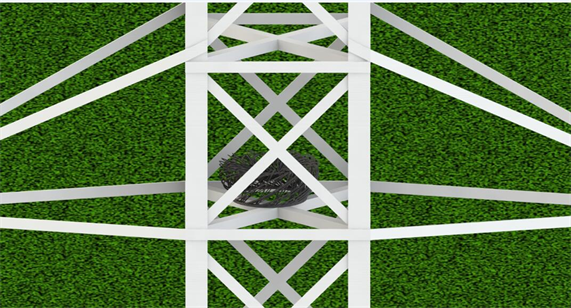Figure 12. Schematic diagram of bird’s nest vertical shooting angle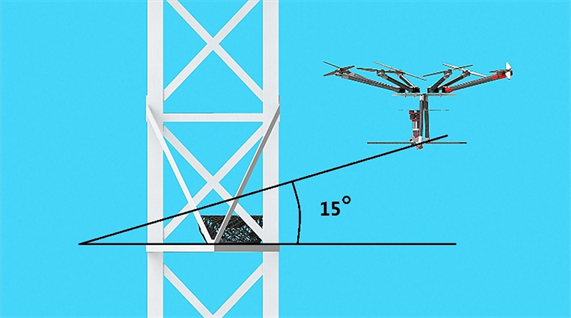Figure 13. Schematic diagram of bird’s nest vertical shooting angle

4.3. 人工巡检和无人机巡检的对比

1) 对于杆塔上的鸟巢而言，无人机的摄像头的识别能力和人的识别能力基本一致：

2) 人工巡检的视角为自下而上，故背景为蓝天，而无人机的视觉为由上而下，故背景大部分为绿色植被。(a)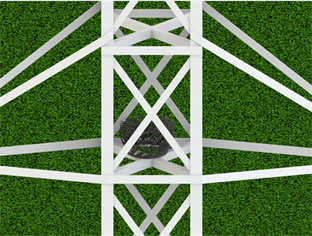(b)

Figure 14. Inspection comparison chart

5. 结论

NOTES

*通讯作者。

  易辉, 熊幼京, 周刚, 等. 架空输电线路鸟害故障分析及对策[J]. 电网技术, 2008, 32(20): 95-100.  朱先志. 输电线路鸟害规律分析及防治对策[J]. 华中电力, 2000, 13(6): 47-49.  王少华, 叶自强. 架空输电线路鸟害故障及其防治技术措施[J]. 高压电器, 2011, 47(2): 61-67.  全晓, 王坤, 段君丽. 架空输电线路鸟害故障及其防治技术措施[J]. 科技与企业, 2015(9): 245-245.  巢亚锋, 徐志强, 岳一石, 等. 湖南输电线路鸟害故障特征分析及防范措施[J]. 高电压技术, 2016, 42(12): 3853-3860.  王允杰. 发现率与输电线路的巡视[J]. 吉林电力, 1982(6): 28-33.  杨可. 超高压输电线路运行可靠性实时监测理论[D]: [硕士学位论文]. 成都: 四川大学, 1996.  陈坤. 鸟害是输电线路难以防治的重点[J]. 中小企业管理与科技, 2008(27): 113-114.  徐晶, 韩军, 童志刚, 等. 一种无人机图像的铁塔上鸟巢检测方法[J]. 计算机工程与应用, 2017, 53(6): 231-235.  陈晓兵, 马玉林, 徐祖舰. 无人飞机输电线路巡线技术探讨[J]. 南方电网技术, 2008, 2(6): 59-61.  李力. 无人机输电线路巡线技术及其应用研究[D]: [硕士学位论文]. 长沙: 长沙理工大学, 2012.  刘代志, 刘志刚, 李夕海. 无人机情报信息处理中的图像处理技术[C]//信号与信息处理技术信号与信息处理全国联合学术会议. 北京: 北京航空航天大学出版社, 2005: 144-147.  Todt, E. and Torras, C. (2005) Color-Contrast Landmark Detection and Encoding in Outdoor Images. In: Computer Analysis of Images and Patterns, Springer, Berlin Heidelberg, 612-619. https://doi.org/10.1007/11556121_75  Angulo, J. and Serra, J. (2003) Color Segmentation by Ordered Merg-ings. International Conference on Image Processing, Vol. 3, II-125-8.  胡焯源, 曹玉东, 李羊. 基于HSV颜色空间的车身颜色识别算法[J]. 辽宁工业大学学报(自然科学版), 2017(1): 10-12.  高程程, 惠晓威. 基于灰度共生矩阵的纹理特征提取[J]. 计算机系统应用, 2010, 19(6): 195-198.  侯海苗, 冀小平. 基于灰度共生矩阵的纹理特征[J]. 长治学院学报, 2008, 25(5): 31-32.  Chen, Y. and Yang, F.Y. (2012) Analysis of Image Texture Features Based on Gray Level Co-Occurrence Matrix. Applied Mechanics & Materials, 204-208, 4746-4750. https://doi.org/10.4028/www.scientific.net/AMM.204-208.4746  Liu, L.Y. and Fan, X.J. (2015) The Design of System to Texture Feature Analysis Based on Gray Level Co-Occurrence Matrix. Applied Mechanics & Materials, 727-728, 904-907. https://doi.org/10.4028/www.scientific.net/AMM.727-728.904  Androutsos, D., Plataniotis, K.N. and Venet-sanopoulos, A.N. (1999) A Novel Vector-Based Approach to Color Image Retrieval Using a Vector Angular-Based Distance Measure. Computer Vision & Image Understanding, 75, 46-58. https://doi.org/10.1006/cviu.1999.0767  Androutsos, D. (1999) Efficient Indexing and Retrieval of Color Image Data Using a Vector-Based Approach. National Library of Canada Bibliothèque nationale du Canada.  朱芳芳, 顾宏斌, 孙瑾. 一种改进的Hough变换直线检测算法[J]. 计算机技术与发展, 2009, 19(5): 19-22.  Qin, T., Li, Y., Huang, W., et al. (2009) Image Retrieval Based on Color-Texture Features of Regions for MPEG-7. IEEE International Conference on Network Infrastructure and Digital Content, Beijing, 6-8 November 2009, 456-460. https://doi.org/10.1109/ICNIDC.2009.5360826  Sharma, J., Rai, J.K. and Tewari, R.P. (2014) Co-Occurrence Matrix and Statistical Features as an Approach for Mass Classification. International Conference on Advances in Computing, Communications and Informatics, New Delhi, 24-27 September 2014, 2369-2373. https://doi.org/10.1109/ICACCI.2014.6968364  谢建辉. 纹理特征提取与分类研究[D]: [硕士学位论文]. 武汉: 华中科技大学, 2008.  Popescu, D., Ichim, L., Angelescu, N., et al. (2015) Improving Texture Based Clas-sification of Aerial Images by Fractal Features. International Conference on Control Systems and Computer Science IEEE, Bucharest, 27-29 May 2015, 578-583. https://doi.org/10.1109/CSCS.2015.18  于德明, 沈建, 汪骏, 等. 直升机与人工巡视效果对比分析[J]. 中国电力, 2008, 41(11): 2.